﻿ 20-sim webhelp > Toolboxes > Mechatronics Toolbox > Servo Motor Editor > Theory > Torque Speed Plot > General Model

# General Model

Navigation:  Toolboxes > Mechatronics Toolbox > Servo Motor Editor > Theory > Torque Speed Plot >

# General ModelThe DC motor model can serve as a prototype model for all permanent magnet motors.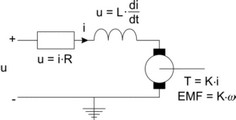Although the models for DC Brushless and AC synchronous motors are slightly different, the DC motor model is still very useful to explain their operation. In this chapter the DC motor model will be used to derive the torque speed plot of a motor and show the use of the torque speed plot for motor selection.

The working principle for all permanent magnet motors is the same: get a constant torque by manipulation of the coil function and the current. The relation between the torque and current is: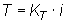The constant KT is generally known as the torque constant. The relation between the induced current and the rotational speed is: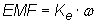The constant Ke is generally known as the voltage constant. For DC motors the torque constant and voltage constant have the same value. Therefore the subscripts T and e are usually omitted. The total voltage can be described as: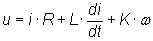If we assume the current is changing only very slowly, the current derivative can be neglected: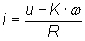With the torque equation: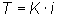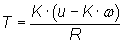The torque at zero speed (stall torque) is found as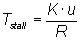which enables us to write the torque as: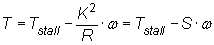where S is commonly know as the steepness. The steepness has many definitions: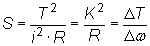The speed where the current is zero (no load speed) is equal to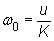The relation between stall torque and no load speed is obvious: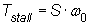which leads to the general torque equation: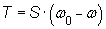This equation can be displayed graphically and is commonly known as the torque speed plot. The graph shows the generated torque as a function of the speed. For constant voltages a straight line from the stall torque to the no load speed is found. The slope of the line is equal to the steepness S.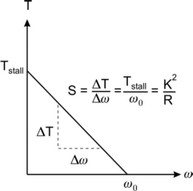The torque speed plot is a useful representation of a motor because we can draw the desired load torque in this plot and to see if the motor is able to produce this torque. In the figure below the load curve is shown in red. It is a constant torque applied to a load, which makes it accelerate until a certain velocity is reached and the torque gets zero.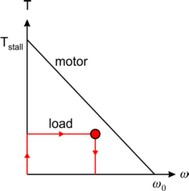The red dot indicates the point of maximum load power. This point is important because it indicates the maximum power that the motor has to deliver.